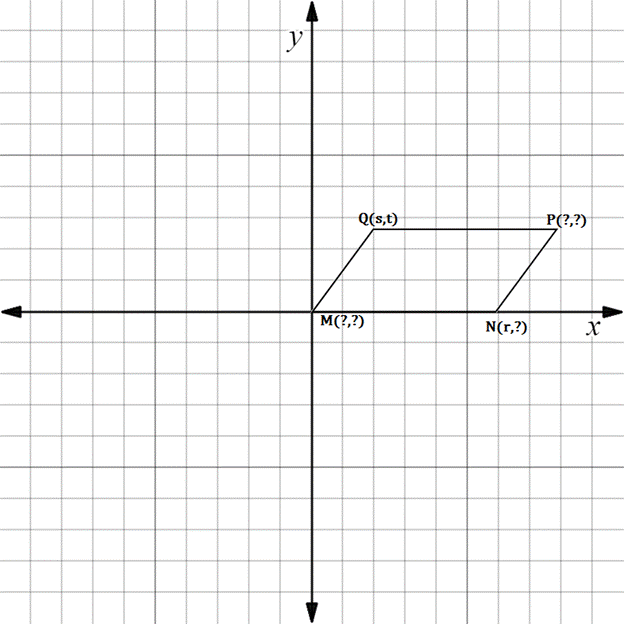Chapter 10.3, Problem 13EElementary Geometry For College St...

7th Edition
Alexander + 2 others
ISBN: 9781337614085

Solutions

Chapter
SectionElementary Geometry For College St...

7th Edition
Alexander + 2 others
ISBN: 9781337614085
Textbook Problem

In Exercises 11 to 16, supply the missing coordinates for the vertices, using as few variables as possible.M N P Q is a parallelogram.

To determine

To find:

The missing coordinates for the vertices.

Explanation

The given figure is shown below.

From the figure, the following observations are made.

Since, the vertex M is at the origin.

The coordinates of M0, 0.

Now, consider the vertex N.

Here, x-coordinate is given as r.

In the N vertex, observe where it lies on y-axis.

Since, it is at 0, the vertex N has the coordinates r, 0.

Consider the vertex P.

In the y-axis, it lies on the same line wher Q lies

Still sussing out bartleby?

Check out a sample textbook solution.

See a sample solution

The Solution to Your Study Problems

Bartleby provides explanations to thousands of textbook problems written by our experts, many with advanced degrees!

Get Started

Find more solutions based on key concepts